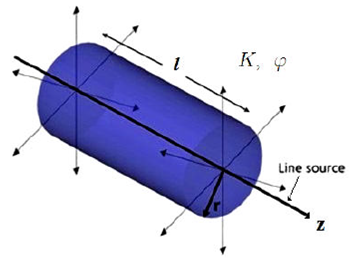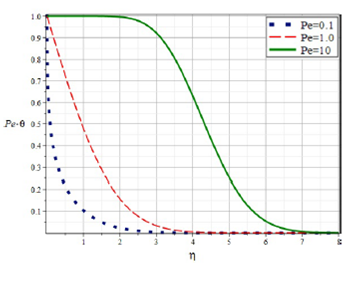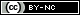## Artigo

•Citado por SciELO
•Acessos

•Similares em SciELO

## versão impressa ISSN 0035-001X

### Rev. mex. fis. vol.67 no.3 México Mai./Jun. 2021  Epub 21-Fev-2022

#### https://doi.org/10.31349/revmexfis.67.365

Research

Fluids Dinamics

Temperature profiles due to continuous hot water injection into homogeneous fluid-saturated porous media through a line source

1ESIME Azcapotzalco, Instituto Politécnico Nacional, Av. de las Granjas 682, Col. Sta. Catarina, Azcapotzalco 02250 CDMX, México. ETSIAE, Universidad Politécnica de Madrid, Plaza Cardenal Cisneros 3, 28040 Madrid, Spain. e-mail: amedinao@ipn.mx

3DCB, Instituto Tecnológico de Querétaro, Av. Tecnológico s/n esq. M. Escobedo, Col. Centro, 76000, Querétaro, Qro., Mexico.

4ESIME Zacatenco, Instituto Politécnico Nacional, Av. Miguel Othon de Mendizabal SN, La Escalera, CDMX, México.

Abstract

We report a theoretical study aimed at determining the temperature distribution of a homogeneous, fluid-saturated porous medium initially at a low temperature, into which a constant flow rate of hot water is injected. The size of the heated region is assumed to be large compared to the radius of the injection pipe, which is idealized as a line source of mass and heat. The temperature distribution is found to be self-similar and to depend on a single dimensionless parameter which is a Peclet number.

Keywords: Heat flow in porous media; flows through porous media; analytical and numerical techniques of heat transfer

PACS 44.30.+v; 47.55.Mh; 44.05.+e

1.Introduction

In this paper, we theoretically determine the temperature distribution of a homogeneous fluid-saturated porous medium due to the injection of hot water through a perforated pipe embedded in the medium. Physically, this is a way of heating the porous matrix and the fluids therein. The problem is ubiquitous in technological areas like heavy and extra-heavy oil recovery 1-6 and for the production and storage of energy in geothermal systems 7-12, among others.

The injection of hot water into a porous medium does imply the simultaneous inflow of mass and thermal energy (enthalpy), both by conduction and advection. In the theoretical treatments, researchers commonly have treated the injection problem as one where the temperature of the injected fluid and the initial temperature of the fluid-saturated porous medium are known, while the spatial and temporal profiles of the temperature of the medium around the injection pipe are computed 1-11.

In the current work, we assume that the hot water has penetrated the porous medium a distance large compared to the radius of the injection pipe, which is then idealized as a line source of mass and heat 13. However, we neglect the effects of gravity, which would come into play at later times. This leaves aside buoyancy-driven flows in miscible and immiscible fluids 14. Such cases correspond, for instance, to the early stages of the injection of hot water into heavy and extra-heavy oil reservoirs (immiscible fluids) and to the injection of hot or cold water into geothermal aquifers (miscible fluids). In these cases, the densities of the fluids involved have similar values, and thermal convection can be assumed to play a secondary role. We also neglect finger-like instabilities.

The basis for the study of problems of hot fluid injection is the energy conservation equation for fluid-saturated porous media. For transient problems, this is a partial differential equation for the temperature as a function of time and the spatial coordinates, which involves advection and conduction heat transfer and initial and boundary conditions that depend on the specific problem considered.

Hot water flooding is a thermal method used in petroleum engineering. It is useful to enhance oil recovery because the high viscosity of the in situ oil drastically decreases when its temperature is increased 1-6. In the case of geothermal systems, cold or hot water can be injected depending on the purpose. Cold water is injected to extract heat from the hot rock by having the water heated before the waterfront reaches the production wells 7-11. Hot water is pumped into shallow permeable layers of rock for seasonal heat storage into reservoirs 12.

In the models, commonly, rock and fluid properties such as the specific heat, density, and thermal conductivity, are considered to be constant in the reservoir. This assumption is valid when the change of temperature in the porous media is small.

The objective of this study is to understand the heat transfer mechanisms in homogeneous porous media through the theoretical modeling of heat transfer coupled with fluid flow. The paper is organized as follows. The physical ingredients of the problem are reviewed in Sec. 2. The mathematical problem is formulated and solved in Sec. 3, showing that the temperature distribution is self-similar and depends on a single dimensionless parameter, a Peclet number that measures the ratio of advection to conduction heat transfer. The features of the temperature profile for slow, medium, and fast injection are discussed. Finally, Sec. 4 summarizes the main conclusions of the work.

2. Physical model

The temperature field around the injection pipe will be analyzed to arrive at conclusions of practical interest.

The hot water to be injected flows through a pipe immersed in the porous medium and leaves the pipe through an array of uniformly distributed orifices in the pipe wall. Owing to the low viscosity of the water, the pressure drop in the pipe can be neglected so that the injection pressure is uniform along the pipe and the flow ensuing in the porous medium can be taken to be two-dimensional in planes perpendicular to the pipe, and purely radial at distances from the pipe large compared to its radius.

The porous medium is homogeneous, with permeability K and porosity φ, and is initially saturated with a quiescent liquid of density nearly equal to the density p of the water and temperature T smaller than the temperature of the hot water in the pipe, Tw. For example, this liquid could be a heavy or extra-heavy oil or fresh/saline water, all of which have densities very similar to the density of the hot water. The liquid is assumed to be immiscible with the injected water at the time scale of the process. We also assume that the fluid and solid phases of the porous medium have the same local temperature (local thermal equilibrium. The pressure in the pipe is such that a constant flow rate of water, q per unit length of the pipe, is injected.

The injected water cools down while moving radially out by transferring heat to the solid matrix and surrounding liquid. The density change of the water during this process is small so that the approximation of constant p is applicable.

3.Analytical model

Figure 1 is a sketch of the problem with the idealizations described in the previous paragraphs. With these idealizations, the radial filtration velocity in the porous medium is v=q/(2πr), where r is the distance to the injection pipe, idealized as a line source at r = 0 (from the continuity equation r-1(rv)/r=0). The radius of the cylindrical region occupied by the injected water at a time t after the onset of injection is

rf(t)=qtπ, (1)

from the condition drf/dt=v(r=rf) with rf(0)=0.Figure 1 Schematic of the hot water radial injection through a unit length, l, of a line source into a homogeneous porous medium of permeability K and porosity φ. The flow rate of liquid injected per unit length of the source, q, and the heat injected per unit length of the source per unit time, Φ, are specified.

The temperature of the porous medium obeys the energy equation 10,15,16

(ρc)mTt+(ρc)fq2πrTr=1rrrkmTr, (2)

where (ρc)f is the volumetric heat capacity of the liquid and the properties of the solid matrix, and the liquid are integrated into the average volumetric heat capacity and thermal conductivity

(ρc)m=(1-φ)(ρc)s+φ(ρc)fandkm=(1-φ)ks+φkf. (3)

These magnitudes will, in general, be discontinuous at r=rf. In what follows, to simplify the analysis, the values of the volumetric heat capacity and thermal conductivity are taken to be the same for both liquids. Removing this assumption does not affect the self-similar character of the solution discussed in the remainder of the paper.

Defining

q˜=ρcfρcmq (4)

and writing (2) in conservative form, we have

Tt+1rrq˜2πT-αmrTr=0, (5)

where αm=km/(ρc)m is the thermal diffusivity of the rock/liquid system.

The quantity (ρc)f/(ρc)fv would be the velocity of the thermal front in the absence of heat conduction 17. Since typically (ρc)s>(ρc)f, this front would lag behind the liquid front r = r f 17,18.

Equation (5) must be solved with the initial and boundary conditions

T=Tatt=0q˜2π(T-T)-αmrTr=Φ2π(ρc)mforr0T=Tforr (6)

where Φ is the heat injected per unit time per unit length of the source, which is taken to be constant (see comments following Eq. (9) later in this section) and appears as the sum of the advection and conduction fluxes in the medium 19.

The solution of (5) and (6) is self-similar, of the form

T-T=Φ2πkmθ(η)withη=rαmt. (7)

Carrying this to (5) and (6), we find

θ''+1-Peη+η2θ'=0withPeθ-ηθ'=1forη0andθ=0forη, (8)

where Pe=q˜/(2παm) and primes denote differentiation with respect to η.

The solution of (8) is

θ=1Pe1-12Pe-1ΓPe20ηηPe-1e-14η2dη, (9)

which is plotted in Fig. 2 for three values of the Peclet number Pe. As can be seen, θ1/Pe for η0 in all the cases. The conduction heat flux tends to zero when r0, and the injected heat Φ can be directly related to the temperature Tw of the water in the injection pipe: Φ=(ρc)fq(Tw-T). Notice that this relation and the pressure variations that Darcy’s law associates to the radial filtration velocity are time-independent. This justifies the assumption of constant values of the injected flow rate and Φ in realistic cases.Figure 2 The dimensionless quantity Pe θ as a function of the similarity variable η given by Eq. (9). The blue dotted curve corresponds to Pe = 0:1, the red dashed curve to Pe = 1, and the green continuous-curve is for Pe = 10. All curves start at Pe θ = 1 for η = 0.

The figure shows three very different behaviors for the quantity Peθ by changing the Peclet number, by an order of magnitude each time: the blue dotted curve is for Pe=0.1, which means that heat diffusion dominates over the advection of the flow, the red dashed curve for Pe = 1 shows the case where advection and diffusion have the same intensity and the green-continuous curve corresponds to Pe = 10, which means that advection dominates strongly on conduction.

The importance of the dimensionless plot in Fig. 2 is that it shows different ways of cooling down the injected hot fluid in the porous medium, depending primarily on the value of the Peclet number. For instance, a low value of Pe (which can be given as Pe=ρcfq/2πkm) means that injection of the hot liquid is slow and/or that its volumetric heat capacity is small, and/or conversely, the average thermal conductivity of the water-saturated porous medium is high.

In the context of oil recovery, sand (unconsolidated) 1 2 and sandstone (consolidated) 4 reservoirs, which store large reserves of heavy and extra-heavy oil, have been exploited by using hot water injection. In these cases, the Peclet number can be determined using order-of-magnitude estimates as follows: the average thermal conductivity k m is, in both cases, in the order of 1 W/m K in a wide range of temperatures 20,21, meaning that the heat transfer from the water to the porous matrix is very efficient. The volumetric heat capacity of water (ρc)f is in the order of 1 MJ/(m3K)22). Consequently, the ratio (ρc)f/km is of order 106s/m2, and it is found that for water flooding into this type of reservoir, the best form of change Pe is by changing the injection rate. As an example, if a process requires Pe10-1 then q10-7m2/s, and so forth and so on for the other Peclet numbers. Geothermal aquifers have similar values of the ratio (ρc)f/km 23 and, therefore, similar conclusions as aforementioned for Pe and q are reached.

4.Conclusions

An analysis has been carried out of the heating of an unbounded porous medium by injection of hot water through a perforated pipe. After a short initial period that is not analyzed, the size of the heated region becomes large compared to the radius of the injection pipe, which can be idealized as a line source of mass and heat. On the other hand, the temperature distribution is axisymmetric before buoyancy comes into play. When both conditions are satisfied, the solution is self-similar and depends only on a Peclet number. This solution has been computed, and its dependence on the Peclet number has been discussed. In conditions typical of hot water injection into the sand and sandstone heavy oil reservoirs, the ratio of volumetric heat capacity of water to the average thermal conductivity of the medium is very large, and the Peclet number is essentially a measure of the flow rate of water injected, which determines the way the thermal energy behaves during the injection.

Acknowledgments

A.M. acknowledges support from IPN, from Fondo de Hidrocarburos-CONACYT, and from Universidad Politécnica de Madrid through the project “Fundamental models for the thermal methods of steam injection in EOR”. He also appreciates the invaluable support of Prof. A. Liñan, who guided him in part of this work. Finally, the authors are grateful to an anonymous reviewer whose comments on an earlier version of the manuscript helped us to clarify it and to improve the presentation of the solution in Eq. (9).

1. H. A. Lauwerier, Appl. Sci. Res. 5 (1955) 145. https://doi.org/10.1007/BF03184614 [ Links ]

2. A. G. Spillette, Jour. Can. Petr. Tech. 4 (1965) 213. https://doi.org/10.2118/65-04-06 [ Links ]

3. K. Yu, G. Zhao, Modeling of heat transfer coupled with fluid flow for temperature transient analysis during SAGD process, SPE Latin America and Caribbean Heavy and Extra Heavy Oil Conference, SPE-181208-MS 2016. [ Links ]

4. M. I. Miah, M. A. Elhaj, S. Ahmed, M. E. Hossain, Fuel 226(2018) 423. And references therein. https://doi.org/10.1016/j.fuel.2018.04.018 [ Links ]

5. N. Chevarunotai, A. R. Hasan, C. S. Kabir, R. Islam, Jour. Petrol. Explor. Prod. Techol. 8 (2018) 799. https://doi.org/10.1007/s13202-017-0397-0 [ Links ]

6. Z. Wu, H. Liu, Jour. Petrol. Explor. Prod. Techol. 9 (2019) 1547.https://doi.org/10.1007/s13202-018-0568-7 [ Links ]

8. A. W. Woods, S. D. Fitzgerald, Jour. Fluid Mech. 343 (1997)303. https://doi.org/10.1146/annurev.fluid.31.1.171 [ Links ]

9. A. W. Woods, Annu. Rev. Fluid Mech 31 (1999) 171. https://doi.org/10.1146/annurev.fluid.31.1.171 [ Links ]

10. A. W. Woods, Flow in Porous Rocks: Energy and Environmental Applications (Cambridge University Press, Cambrige 2015). [ Links ]

11. S. Ganguly, M. S. Mohan Kumar, Hydrogeol. Jour. 22 (2014) 351. https://doi.org/10.1007/s10040-013-1048-2 [ Links ]

12. P. Dudfield, A. W. Woods, Jour. Fluid Mech. 707 (2012) 467. https://doi.org/10.1017/jfm.2012.291 [ Links ]

13. V. N. Kurdyumov, A. Liñan, Jour. Fluid Mech. 427 (2001) 389. https://doi.org/10.1017/S0022112000002482 [ Links ]

14. S. S. Gopalakrishnan, Jour. Fluid Mech. 892 (2020) A13. https://doi.org/10.1017/jfm.2021.42 [ Links ]

15. O. M. Phillips, Geological fluid dynamics: Sub-surface flow and reactions (Cambridge: Cambridge University Press, Cambridge 2009). [ Links ]

16. D. A. Nield, A. Bejan, Convection in porous media (Springer, New York 2013). [ Links ]

17. A. Woods, S.D. Fitzgerald, J. Fluid Mech. 251 (1993) 563. https://doi.org/10.1017/S0022112093003520 [ Links ]

18. T. Menand, A. Raw, A. W. Woods, Geophys. Res. Lett. 30 (2003) 1291. https://doi.org/10.1029/2002GL016294 [ Links ]

19. R. A. Wooding, Jour. Fluid Mech. 15 (1963) 527. https://doi.org/10.1017/S0022112063000434 [ Links ]

20. Z. Abdulagatova, I.M. Abdulagatov, V.N. Emirov, Int. Jour. Rock Mech. Min. Sci. 46 (2009) 1055. https://doi.org/10.1021/je050016a [ Links ]

21. M. Labus, K. Labus, Jour. Therm. Anal. Calorim. 132 (2018) 1669. https://doi.org/10.1007/s10973-018-7090-5 [ Links ]

22. S. Gehlin, Borehole thermal energy storage, in Advances in Ground-Source Heat Pump Systems, S. Rees (Ed.) 295-327 (Woodhead Publishing, Duxford, UK, 2016). doi:10.1016/b978-0-08-100311-4.00011-x [ Links ]

23. V. Wagner, P. Bayer, G. Bisch, M. Kübert, Ph. Blum, Water Resour. Res. 50 (2014) 71. https://doi.org/10.1002/2013WR013939 [ Links ]

Received: July 09, 2020; Accepted: February 08, 2021Este es un artículo publicado en acceso abierto bajo una licencia Creative Commons